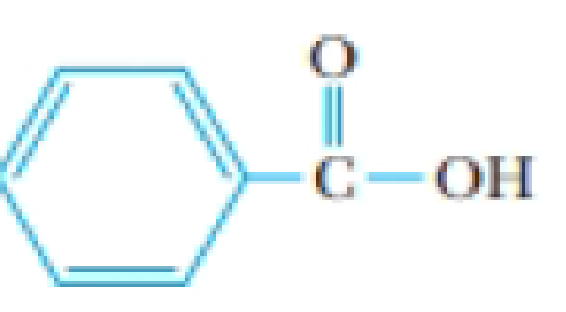Chapter 13, Problem 87IL

Chapter
Section
Textbook Problem

A solution of benzoic acid in benzene has a freezing point of 3.1 °C and a boiling point of 82.6 °C. (The freezing point of pure benzene is 5.50 °C, and its boiling point is 80.1 °C) The structure of benzoic acid isBenzoic acid, C6H5CO2HWhat can you conclude about the state of the benzoic acid molecules at the two different temperatures? Recall the discussion of hydrogen bonding in Section 11.3.

Interpretation Introduction

Interpretation: The state of benzoic acid at two different temperatures has to be determined.

Concept introduction:

Colligative properties: Properties of solutions which having influence on the concentration of the solute in it. Colligative properties are,

• Decrease in the vapor pressure
• Increase in the boiling point
• Decline in the freezing point
• Osmotic pressure

Boiling point elevation: The boiling point of the solution varies with the solute concentration.

Elevation in boiling point = ΔTbp= Kbp. msolute,where,Kbp=molal boiling point elevation constant,msolute=molality of solute

Freezing point depression: The freezing point of the solution varies with the solute concentration.

Freezing point depression = ΔTfp= Kfp. msolute,where,Kfp=molal freezing point depression constant,msolute= molality of solute.

Molality (m): Molality is the number of moles of solute present in one kilogram of solvent.

Molality (m) =Numberofmolesofsolute1kgofsolvent

Explanation

The molality of benzoic acid at two different temperatures is calculated.

Given,

Freezingpointofbenzene=5.5oCFreezingpointofsolution=3.1oCΔT=2.40C

Boilingpointofbenzene=80.1oCBoilingpointofsolution=82.6oCΔT=2.50C

Molal freezing point depression constant of benzene is 5.120C/m

Molal boiling point elevation constant of benzene is 2.530C/m

Change in freezing point is calculated by using the equation,

ΔTfp=Kfpmsolute

Hence,

The concentration is calculated by,

Concentration,msolute=ΔTfpKfp=(2

Still sussing out bartleby?

Check out a sample textbook solution.

See a sample solution

The Solution to Your Study Problems

Bartleby provides explanations to thousands of textbook problems written by our experts, many with advanced degrees!

Get Started

6.10 Define the term refraction.

Chemistry for Engineering Students

Define the following terms: a. chromosome b. chromatin

Human Heredity: Principles and Issues (MindTap Course List)

What is a peptide bond, and what type of reaction forms it?

Biology: The Dynamic Science (MindTap Course List)

What are the sources of compounds that undergo gluconeogenesis?

Chemistry for Today: General, Organic, and Biochemistry